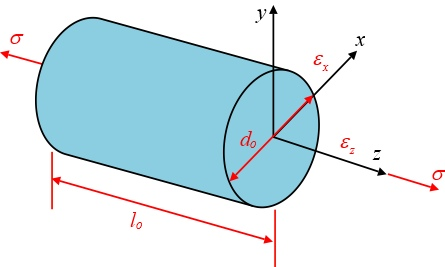# A cylindrical metal specimen 10.7 mm in diameter and 95 mm long is to be subjected to a tensile...

## Question:

A cylindrical metal specimen 10.7 mm in diameter and 95 mm long is to be subjected to a tensile force of 300 N where the resulting deformation is totally elastic.

(a) If the final length must be less than 95.04 mm, compute the strain {eq}{{\varepsilon }_{z}} {/eq} and the young's modulus for this specimen?

(b) What is the strain, {eq}{{\varepsilon }_{x}} {/eq}, in the x-direction (due to the reduction in diameter), given the conservation of mass? What is the Poisson's ratio given

{eq}\nu =-\frac{{{\varepsilon }_{x}}}{{{\varepsilon }_{z}}} {/eq}## Poisson's Ratio:

The proportion of the lateral strain (contraction) to the longitudinal strain (expansion), which occur due to the load applied on the specimen is called Poisson's Ratio. It is expressed as,

{eq}v = \dfrac{{{\varepsilon _{lateral}}}}{{{\varepsilon _{long}}}} {/eq}

Here, {eq}{\varepsilon _{lateral}} {/eq} and {eq}{\varepsilon _{long}} {/eq} are the lateral strain and longitudinal strain respectively.

Given Data:

• The diameter of the specimen is: {eq}d = 10.7\;{\rm{m}} {/eq}
• The length of the specimen is: {eq}l = 95\;{\rm{mm}} {/eq}
• The tensile...

Become a Study.com member to unlock this answer! Create your account